﻿ 火星探测器进入飞行气动测量方法研究
«上一篇文章快速检索 高级检索

 力学学报2015, Vol. 47Issue (1): 8-14  DOI: 10.6052/0459-1879-14-3310

### 引用本文 [复制中英文]

[复制中文]
Yang Lei, Hou Yanze, Zuo Guang, Liu Yan, Guo Bin. AERODYNAMIC CHARACTERISTICS MEASSUREMENT OF MARS VEHICLES DURING ENTRY FLIGHT[J]. Chinese Journal of Ship Research, 2015, 47(1): 8-14. DOI: 10.6052/0459-1879-14-331.
[复制英文]

### 文章历史

2014-10-23收稿
2014-11-26录用
2014-12-12网络版发表

1 气动测量系统模型 1.1 进入飞行任务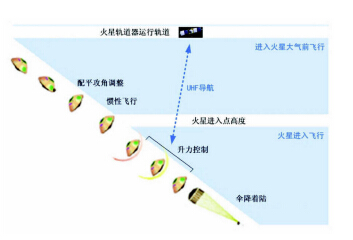图 1 火星探测器进入飞行任务示意图 Fig. 1 Entry flight profile of Mars exploration vehicle
1.2 气动测量系统组成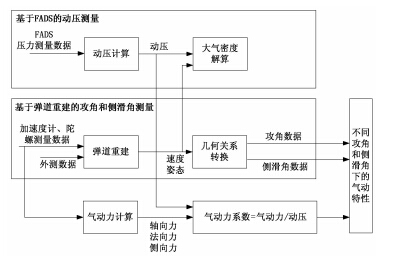图 2 气动测量系统测量方案示意图 Fig. 2 Measurement scheme of aerodynamic characteristics

(2)加速度计：测量进入过程中的气动过载，结合探测器质量，用于获取气动力；

(3)陀螺，测量进入探测器的姿态角速率，用于弹道重建，获取攻角和侧滑角数据.

(4)外测系统：提供探测器的弹道外测位置数据，作为弹道重建的数据基准. 该项数据可通过环火轨道卫星的UHF设备实现.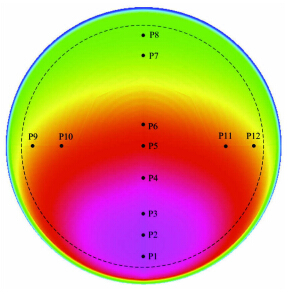图 3 引压孔布局示意图 Fig. 3 Location of pressure measurement points
2 攻角和侧滑角测量方法

 $\dot{\pmb x}_e = f\left({\pmb x}_e ,{\pmb a}_e , {\pmb\omega} _e ,t \right)$ (1)

 ${\pmb Y}_e = {\pmb Y}_m + {\pmb \delta} _d$ (2)

 ${\pmb a } _e = {\pmb a } _m + {\pmb a } _d$ (3)

 ${\pmb \omega }_e = {\pmb \omega }_m + {\pmb \omega}_d$ (4)

 ${\pmb \varTheta} = \left({\pmb Y}_e \left( 0 \right), {\pmb V}_e \left( 0 \right), {\pmb \phi }_e \left( 0 \right), {\pmb a}_d ,{\pmb \omega }_d \right)$ (5)

 ${\pmb\varTheta} _i = {\pmb\varTheta} _{i - 1} + \Delta {\pmb\varTheta} _i$ (6)

 $\Delta {\pmb\varTheta} _i = - \left( {\dfrac{\partial ^2J}{\partial \varTheta _p \varTheta _q }} \right)_{\varTheta _p ,\varTheta _q \in {\pmb\varTheta} }^{ - 1} \left( {\dfrac{\partial J}{\partial \varTheta _p }} \right)_{\varTheta _p \in {\pmb\varTheta} }$ (7)

 $J\left( {\pmb\varTheta} \right) = {\pmb v } {\pmb W }^{ - 1}{\pmb v }^{\rm T} ,\ {\pmb v } = {\pmb Y } _e - {\pmb Y } _m$ (8)

 $\left. \alpha = \arctan \left( {{ - V_y } /{V_x }} \right) \\ \beta = \arcsin \left( {{V_z } / {\left( {V_x^2 + V_y^2 + V_z^2 } \right)^{1 / 2}}} \right) \right\}$ (9)
3 动压测量方法

 $P_i = f_i \left( {\pmb X} \right) + \varepsilon _i = q_c \cos ^2\theta _i + p_\infty + \varepsilon _i$ (10)

 $\cos \left( {{\theta _i}} \right) = \cos \left( \alpha \right)\cos \left( \beta \right)\cos \left( {{\lambda _i}} \right) +\\ \qquad \sin \left( \beta \right)\sin \left( {{\varphi _i}} \right)\sin \left( {{\lambda _i}} \right) + \\ \qquad \sin \left( \alpha \right)\cos \left( \beta \right)\cos \left( {{\varphi _i}} \right)\sin \left( {{\lambda _i}} \right)n$ (11)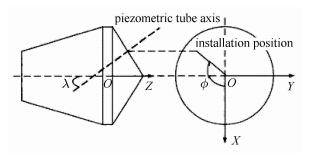图 4 引压孔位置关系示意图 Fig. 4 Location relationship of pressure measurement points

t时刻，n个传感器的测量输出形成下述矢量，
 $\left. \begin{array}{l} P = {\left( {{P_1}\;{P_2}\; \cdots \;{P_n}} \right)^{\rm{T}}}\\ f = {\left( {{f_1}\left( X \right){f_2}\left( X \right)\; \cdots {f_n}\left( X \right)} \right)^{\rm{T}}}\\ \varepsilon = {\left( {{\varepsilon _1}{\rm{ }}{\varepsilon _2}\; \cdots \;{\varepsilon _n}} \right)^{\rm{T}}} \end{array} \right\}$ (12)

 ${\pmb P} = {\pmb f}\left( {\pmb X} \right) + {\pmb \varepsilon }$ (13)

 ${\pmb P} = {\pmb f}\left( {{\pmb X}_0 } \right) + \left( {\dfrac{\partial {\pmb f}}{\partial {\pmb X}}} \right)_{{ X} = { X}_0 } \cdot \Delta {\pmb X} + {\pmb \varepsilon }$ (14)

 ${\pmb H} = \left( {\dfrac{\partial {\pmb f}}{\partial {X}}} \right)_{{X} = {X}_0 }$ (15)

 ${\pmb y} = {\pmb P} - {\pmb f}\left( {{\pmb X}_0 } \right)$ (16)

 ${\pmb y} ={\pmb H} \cdot \Delta {\pmb X} + {\pmb \varepsilon }$ (17)

 $\Delta {\pmb X} = \left( {{\pmb H}^{\rm T}{\pmb S}^{ - 1}{\pmb H}} \right)^{ - 1}{\pmb H}^{\rm T}{\pmb S}^{ - 1} {\pmb y}$ (18)

 ${\pmb S}_E = \left(\!\!\begin{array}{ccccc} {\sigma _1^2 } & 0 & \cdots & \cdots & 0 \\ 0 & {\sigma _2^2 } & 0 & \cdots & \vdots \\ \vdots & 0 & \ddots & 0 & \vdots \\ \vdots & \vdots & 0 & {\sigma _{n - 1}^2 } & 0 \\ 0 & 0 & \cdots & 0 & {\sigma _n^2 } \end{array}\!\! \right)$ (19)

 $\begin{array}{l} R = \frac{{{p_\infty }}}{{{q_c} + {p_\infty }}} = {\left[ {\frac{2}{{\left( {\gamma + 1} \right)M{a^2}}}} \right]^{{\textstyle{\gamma \over {\gamma - 1}}}}} \cdot \\ \qquad {\left[ {\frac{{2\gamma M{a^2} - \left( {\gamma - 1} \right)}}{{\left( {\gamma + 1} \right)}}} \right]^{{\textstyle{1 \over {\gamma - 1}}}}},\\ \gamma = 1.335,\;Ma > 1 \end{array}$ (20)

 $q_\infty = \dfrac{\gamma }{2}\left( {q_c + p_\infty } \right) R Ma^2$ (21)

4 仿真分析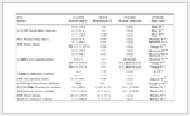表 1 火星探测进入飞行任务总体参数 Table 1 Parameters of entry flight mission表 2 气动测量系统方案仿真参数 Table 2 Simulation parameters of aerodynamics measurement system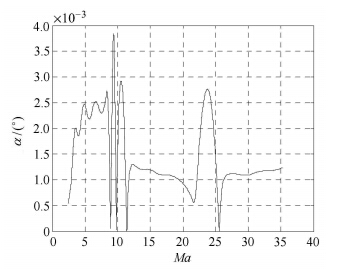图 5 基于弹道重建的攻角测量精度 Fig. 5 Angle of attack error based on trajectory reconstruction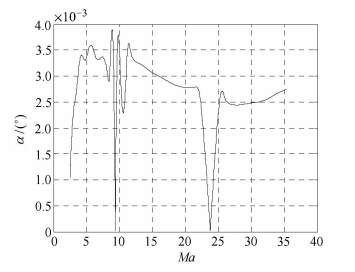图 6 基于弹道重建的侧滑角测量精度 Fig. 6 Angle of sideslip error based on trajectory reconstruction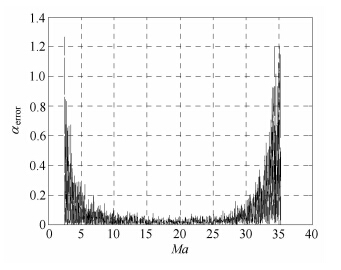图 7 基于FADS测量的攻角测量精度 Fig. 7 Angle of attack error based on FADS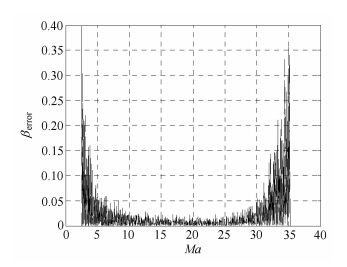图 8 基于FADS测量的侧滑角测量精度 Fig. 8 Angle of sideslip error based on FADS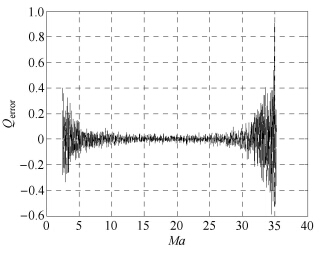图 9 动压精度水平 Fig. 9 Dynamic pressure error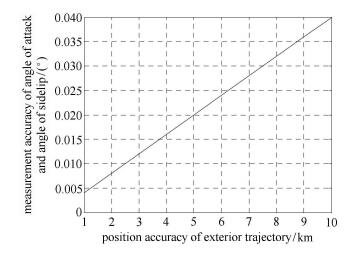图 10 不同外测精度下的攻角和侧滑角测量精度 Fig. 10 Angle of attack and angle of sideslip error on different trajectory measurement error

5 结 论

  Gazarik MJ, Wright MJ. Overview of the MEDLI project. IEEE 2008 Aerospace Conference, 2008  Christopher DK, Prasad K, Mark S, et al. Mars entry atmospheric data system trajectory reconstruction algorithms and flight results. AIAA 2013-0028, 2003  Euler EA, Adams GL, Hopper FW. Design and reconstruction of the viking lander descent trajectories. Journal of Guidance and Control, 1978, 1(5): 372-378  Spencer DA, Blanchard RC, Braun RD, et al. Mars pathfinder entry, descent and landing reconstruction. Journal of Spacecraft and Rockets, 1999, 36(3): 357-366  Blanchard RC, Desai PN. Mars phoenix entry, descent, and landing trajectory and atmosphere reconstruction. Journal of Spacecraft and Rockets, 2011, 48(5): 809-821  Morabito DD. The spacecmn communications blackout problem encountered during passage or entry of planetary atmospheres. Pasadena, Califomia: Jet Propulsion Laboratory, August, 2002  Edwards CD, Bell DJ, Gladden RE, et al. Relay support for the Mars science laboratory mission. Pasadena, Califomia: Jet Propulsion Laboratory, March, 2013  Jean FL, Jean L. Innovative navigation schemes for state and parameter estimation during Mars entry. Journal of Guidance, Control & Dynamics, 2007, 30(1): 169-184  Glenn L, Andreas EM, Daniel B. Real-time navigation for Mars missions using the Mars network. Journal of Spacecraft and Rockets, 2008, 45(3): 519-533  崔平远, 于正湜, 朱圣英. 火星进入段自主导航技术研究现状与展望. 宇航学报, 2013, 34(4): 443-456 (Cui Pingyuan, Yu Zhengshi, Zhu Shengying. Research progress and prospect of autonomous navigation techniques for Mars entry phase. Journal of Astronautics, 2013, 34(4): 443-456 (in Chinese))  汪清, 和争春, 方方等. 返回舱弹道重建与黑障区弹道再现研究. 宇航学报, 2004, 25(6): 595-615 (Wang Qing, He Zhengchun, Fang Fang, et al. Trajectory reconstruction and black-out-area trajectory makeup for reentry capsules of spacecraft. Journal of Astronautics, 2004, 25(6): 595-615 (in Chinese))  王文强. 火星探测中进入弹道与大气模型重建. 航天返回与遥感, 2013, 34(2): 8-15, (Wang Wenqiang. Atmospheric entry trajectory and atmosphere model reconstruction in Mars exploration. Spacecraft Recovery and Remote Sensing, 2013, 34(2): 8-15 (in Chinese)
AERODYNAMIC CHARACTERISTICS MEASSUREMENT OF MARS VEHICLES DURING ENTRY FLIGHT
Yang Lei, Hou Yanze, Zuo Guang, Liu Yan, Guo Bin
Institute of Manned Space System Engineering, Beijing 100094, China
Fund: The project was supported by the National Natural Science Foundation of China (61403028)
Abstract: Performing aerodynamic measurement can acquire a mounts of data during Mars entry exploration. This work benefits for aerodynamic characteristics confirmation and improves key flight performance, such as landing accuracy. In this study, an aerodynamic measurement system scheme is proposed based on trajectory reconstruction and flush air data system. Angle of attack and angle of side slip are measured by output error method with measured trajectory; dynamical pressure is measured via Flush air data system and least square optimal estimation. By simulation, it shows that the proposed measurement scheme has high measure accuracy, and dynamical pressure accuracy is 1%, and angle of attack and angle of side slip accuracy are improved more than one time. This study is helpful for aerodynamic measurement of deep-space exploration entry flight, such as Mars exploration entry flight.
Key words: Mars exploration    entry    flush air data system    aerodynamic characteristics measurement